## Dispersion

 7.1 Dispersion

 Some formula \begin{aligned} &\text{Size of class interval}= \frac{\text{Largest data value - Smallest data value}}{\text{Number of classes}} \end{aligned} \begin{aligned} &\text{Lower boundary}=\frac{\text{Upper limit of the class before it + Lower limit of the class}}{2} \end{aligned} \begin{aligned} &\text{Upper boundary}=\frac{\text{Upper limit of the class + Lower limit of the class after it}}{2} \end{aligned} \begin{aligned} &\text{Midpoint}=\frac{\text{Lower limit + Upper limit}}{2} \end{aligned}

 Example 1 The data below shows the heights, to the nearest cm, of a group of Form 5 pupils. \begin{aligned} \begin{matrix} 153 & 168 &163 &157\\ 158 & 161 &165 &162\\ 145 &150 &158& 156\\ 166 &163 &152& 155\\ 158 &173& 148 &164\\ \end{matrix} \end{aligned} a) Determine the class intervals for the data, if the number of classes required is 6. b) Construct a frequency table based on the information in a). Hence, complete the frequency table with the lower limit, upper limit, midpoint, lower boundary and upper boundary. Solution: \begin{aligned} \text{a) }&\text{The largest data is 173 and the smallest data is 145.}\\ &\text{If the number of classes is 6, then the size of each class interval}\\ &=\frac{173}{145}\\ &=4.7\approx 5.\\ &\therefore\text{The class intervals are 145 – 149, 150 – 154, 155 – 159,}\\&\text{ 160 – 164, 165 – 169 and 170 – 174.} \end{aligned} b)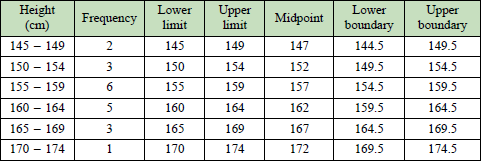What is cumulative frequency? The cumulative frequency of a class interval is the sum of the frequency of the class and the total frequency of the classes before it. This gives an ascending cumulative frequency.

Example 2

Construct a cumulative frequency table from the frequency table below.

 Age 10-19 20-29 30-39 40-49 50-59 Frequency 4 5 9 8 5

Solution:

 Age Frequency Cumulative frequency 10-19 4 4 20-29 5 9 30-39 9 18 40-49 8 26 50-59 5 31

 What is histogram? Histogram is a graphical representation in which the data is grouped into ranges by using contiguous bars. The height of the bar in histogram represents the frequency of a class. Steps for constructing a histogram: Find the lower boundary and upper boundary of each class interval. Choose an appropriate scale on the vertical axis. Represent the frequencies on the vertical axis and the class boundaries on the horizontal axis. Draw bars that represent each class where the width is equal to the size of the class and the height is proportionate to the frequency.

 What is frequency polygon? A frequency polygon is a graph that displays a grouped data by using straight lines that connect midpoints of the classes which lie at the upper end of each bar in a histogram. Steps for constructing a frequency polygon: Mark the midpoints of each class on top of each bar. Mark the midpoints before the first class and after the last class with zero frequency. Draw straight lines by connecting the adjacent midpoints.

Example 3

The frequency table below shows the speed of cars in $$\text{km h}^{-1}$$, recorded by a speed trap camera along a highway in a certain duration. Represent the data with a histogram and frequency polygon by using a scale of 2 cm to 10 $$\text{km h}^{-1}$$ on the horizontal axis and 2 cm to 10 cars on the vertical axis.

 Speed $$(\text{km h}^{-1})$$ 70-79 80-89 90-99 100-109 110-119 120-129 Number of cars 5 10 20 30 25 10

Solution: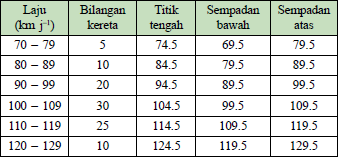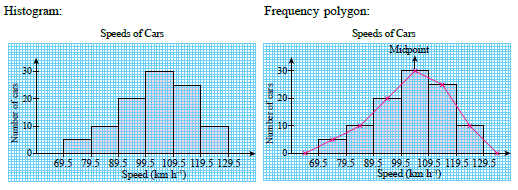Distribution shapes of data When describing a grouped data, it is important to be able to recognise the shapes of the distribution. The distribution shapes can be identified through a histogram or frequency polygon. Common distribution shapes are as follows: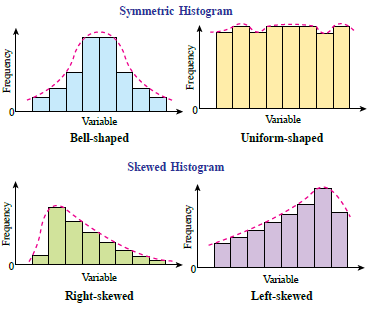What is an ogive? A cumulative frequency graph, also known as an ogive. When the cumulative frequencies of a data are plotted and connected, it will produce an S-shaped curve. Ogives are useful for determining the quartiles and the percentiles. Steps for constructing an ogive: Add one class before the first class with zero frequency. Find the upper boundary and the cumulative frequency for each class. Choose an appropriate scale on the vertical axis to represent the cumulative frequencies and the horizontal axis to represent the upper boundaries. Plot the cumulative frequency with the corresponding upper boundary. Draw a smooth curve passing through all the points.

 Quartile For a grouped data with number of data $$N$$, the quartiles can be determined from the ogive. $$Q_1$$, $$Q_2$$ and $$Q_3$$ are the values that correspond to the cumulative frequency \begin{aligned} \frac{N}{4} \end{aligned}, \begin{aligned} \frac{N}{2} \end{aligned} and \begin{aligned} \frac{3N}{4} \end{aligned} respectively.

 Example 4 The frequency table below shows the salt content of 60 types of food. a) Construct an ogive to represent the data. b) From your ogive, determine (i) the first quartile (ii) the median (iii) the third quartile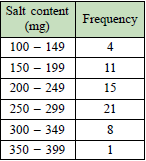Solution: a)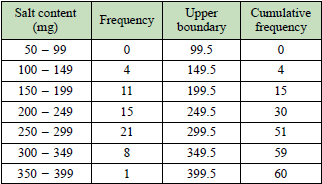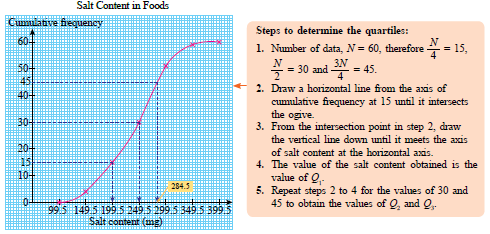b) \begin{aligned} &\frac{1}{4}\times 60 = 15\\ &\text{From the graph, the first quartile,}\\ &Q_1 = 199.5 \text{ mg}.\\\\ &\frac{1}{2}\times 60 = 30\\ &\text{From the graph, the median,}\\ &Q_2 = 249.5 \text{ mg}\\\\ &\frac{3}{4}\times 60 = 45\\ &\text{From the graph, the third quartile,}\\ & Q_3 = 284.5 \text{ mg} \end{aligned}

 Percentile A percentile is a value that divides a set of data into 100 equal parts and is represented by $$P_1,P_2,P_3,\dots,P_{99}$$.